# Physics - Classical Mechanics - Angular Momentum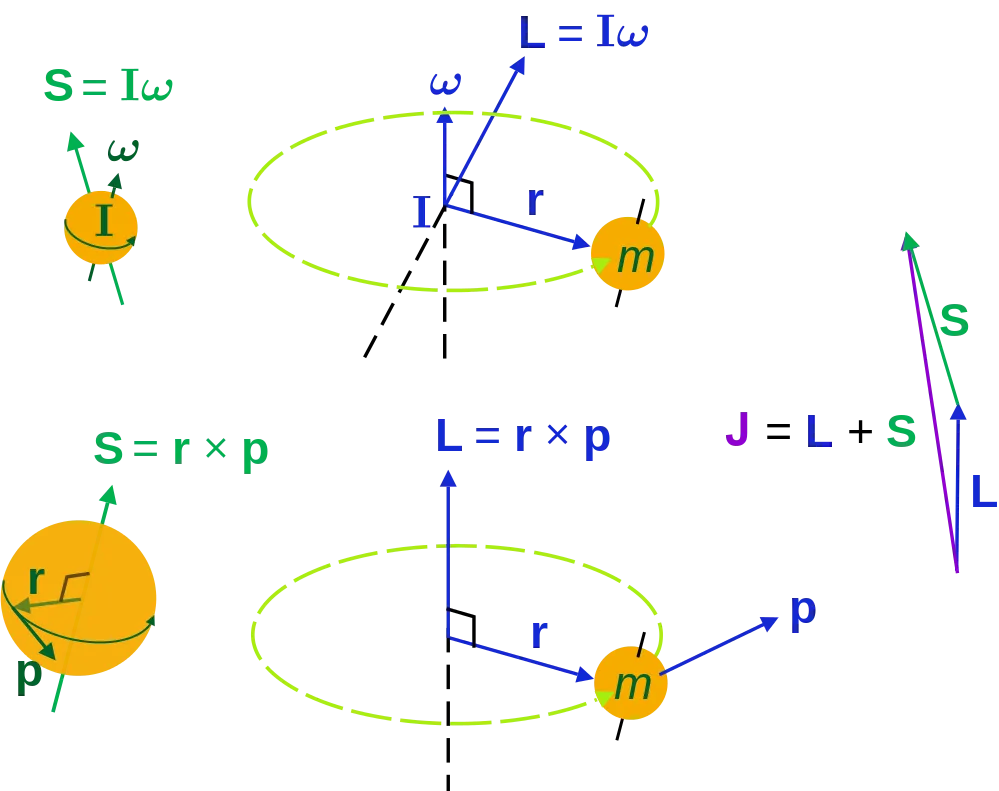[Image 1]

## Introduction

Hey it's a me again @drifter1! Today we continue with Physics and more specifically the branch "Classical Mechanics" to get into Angular Momentum and it's Conservation. So, without further ado, let's get straight into it!

## Angular Momentum

Similarly to how we defined momentum for Translational motion (p = m·v), we also define a different kind of momentum, called Angular Momentum, for Angular motion! This quantity characterizes the rotary inertia of an object or system of objects that's rotating about an axis. It's symbolized by the capital letter 'L'. Thinking about the relationship of angular and linear motion, we can also define angular momentum as the product of linear momentum times the perpendicular distance from the axis of rotation: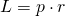Substituting p with it's corresponding part m·v we get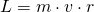Knowing that the tangential velocity can be written in respect to angular velocity as v = ω·r we can substitute v to obtain: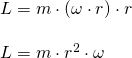The first part corresponds to the moment of inertia I and so we finally have:We can see that angular momentum is calculated in Kg · m2/s. Of course, Angular momentum is a vector quantity pointing in the same direction as the angular velocity. Furthermore, notice that this equation tells us that objects with large moments of inertia (Ex. Earth) or large angular velocity (Ex. centrifuge) have a very large angular momentum! Quite useful to know :)

## Conservation of Angular Momentum

Similar to linear momentum, angular momentum is also being conserved! As long as no external torque acts on a system (net τ = 0), the total angular momentum of the system remains constant. Which means that I·ω = constant. From the article about torque and it's connection to angular acceleration we have: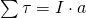Substituting a with the equation that connects it with ω (a = dω/dt) we have:So, the torque acting on a system is equal to the rate of change of angular momentum. When no external torque acts on the system (τ = 0) we have:
Which shows us that the angular momentum in some state 1 is equal to the angular momentum in some state 2, because the angular momentum remain constant (dL/dt = 0).

Again no examples today, as examples around the whole topic of angular motion will come in a follow-up article ;)

## RESOURCES:

### References

1. https://www.britannica.com/science/angular-momentum
2. https://www.sciencetopia.net/physics/angular-momentum-principles
3. https://opentextbc.ca/physicstestbook2/chapter/angular-momentum-and-its-conservation/

### Images

1.## Final words | Next up

And this is actually it for today's post! Next time we will explain the Physics of Gyroscopes...

See ya!Keep on drifting!

H2
H3
H4
3 columns
2 columns
1 column